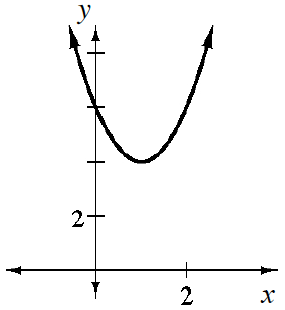### Home > INT3 > Chapter 2 > Lesson 2.2.5 > Problem2-120

2-120.

Sketch the graph of $y=2(x-1)^2+4$.

1. Now write the equation $y=2(x-1)^2+4$ without parentheses.

$y=2x^2−4x+6$

2. What would the difference be between the graphs of the two equations above? This is sort of a trick question; explain your reasoning.

Where is the vertex of $y=2(x−1)^2+4$? Of $y=2x^2−4x+6$? What are their stretch factors?3. What is the parent function of $y=2(x-1)^2+4$?

Refer to the list of parent graphs in the Math Notes box in Lesson 2.2.5.

4. What is the parent function of $y=2x^2-4x+6$?

See part (c).